# Heatmap Themes in Visual Fusion

Heatmaps remain a powerful way of rendering large datasets and provide a great visual indication of distribution (virtually all of the examples here use heatmaps, if you're curious).  In the past, I've gone over some of the inherent visualization benefits of heatmaps, and even a how-to YouTube vid.  But I think a simple list of the pre-packaged heatmap color themes would be a valuable reference (remember, you can always conjure up your own color scheme, just tweak the snippet at the very bottom of this post).  So here they are, lame names and all, mapping the distribution of Target stores...

 Black OutBlue Light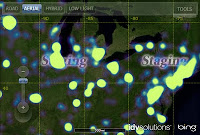Blue Light DiscreteCold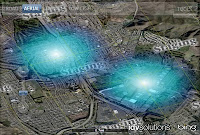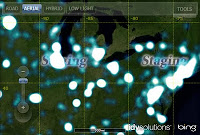For Light Base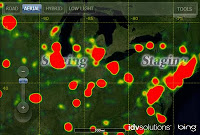Isoline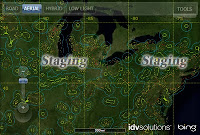NapalmNovaPenguinScorched Earth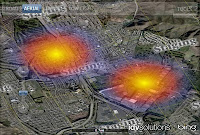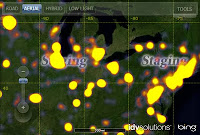Spectrum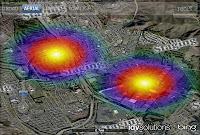Spectrum DiscreteTerrain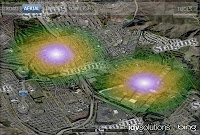WarmWhite Out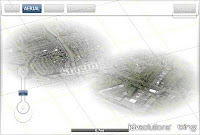Yellow Green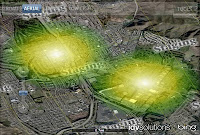Here is a sample heatmap theme that you can tweak if you want to make your own.  The RadialGradientBrush defines the set of color/intensity values and their relative order (0= in the center, 1= at the edge).  The scale parameter lets you shift the relative size of the heatmapping so that you can make the heatmap blobs visible across zoom levels, but this is optional.  You can find some documentation on this stuff here.  Happy heatmapping!

xmlversion="1.0"encoding="utf-8" ?>
<HeatMapThemeName="Napalm">
<Parameters>
<Setname="Illumination"value="False"/>
<Setname="ScaleFactorMappings">
<Setlevel="0"  scale="11.64"/>
<Setlevel="1"  scale="9.31"/>
<Setlevel="2"  scale="7.45"/>
<Setlevel="3"  scale="5.96"/>
<Setlevel="4"  scale="4.77"/>
<Setlevel="5"  scale="3.81"/>
<Setlevel="6"  scale="3.05"/>
<Setlevel="7"  scale="2.44"/>
<Setlevel="8"  scale="1.95"/>
<Setlevel="9"  scale="1.56"/>
<Setlevel="10"scale="1.25"/>
<Setlevel="11"scale="1.00"/>
<Setlevel="12"scale="0.75"/>
<Setlevel="13"scale="0.56"/>
<Setlevel="14"scale="0.42"/>
<Setlevel="15"scale="0.32"/>
<Setlevel="16"scale="0.24"/>
<Setlevel="17"scale="0.18"/>
<Setlevel="18"scale="0.13"/>
<Setlevel="19"scale="0.10"/>
<Setlevel="20"scale="0.08"/>
Set>
Parameters>
HeatMapTheme>